## Work and Energy Review

### Part A: Forced Choice Questions

1. Which of the following statements are true about work? Include all that apply.

1. Work is a form of energy.
2. A Watt is the standard metric unit of work.
3. Units of work would be equivalent to a Newton times a meter.
4. A kg•m2/s2 would be a unit of work.
5. Work is a time-based quantity; it is dependent upon how fast a force displaces an object.
6. Superman applies a force on a truck to prevent it from moving down a hill. This is an example of work being done.
7. An upward force is applied to a bucket as it is carried 20 m across the yard. This is an example of work being done.
8. A force is applied by a chain to a roller coaster car to carry it up the hill of the first drop of the Shockwave ride. This is an example of work being done.
9. The force of friction acts upon a softball player as she makes a headfirst dive into third base. This is an example of work being done.
10. An eraser is tied to a string; a person holds the string and applies a tension force as the eraser is moved in a circle at constant speed. This is an example of work being done.
11. A force acts upon an object to push the object along a surface at constant speed. By itself, this force must NOT be doing any work upon the object.
12. A force acts upon an object at a 90-degree angle to the direction that it is moving. This force is doing negative work upon the object.
13. An individual force does NOT do positive work upon an object if the object is moving at constant speed.
14. An object is moving to the right. A force acts leftward upon it. This force is doing negative work.
15. A non-conservative force is doing work on an object; it is the only force doing work. Therefore, the object will either gain or lose mechanical energy.

2. Which of the following statements are true about power? Include all that apply.

1. Power is a time-based quantity.
2. Power refers to how fast work is done upon an object.
3. Powerful people or powerful machines are simply people or machines which always do a lot of work.
4. A force is exerted on an object to move it at a constant speed. The power delivered by this force is the magnitude of the force multiplied by the speed of the object.
5. The standard metric unit of power is the Watt.
6. If person A and person B do the same job but person B does it faster, then person A does more work but person B has more power.
7. The Newton•meter is a unit of power.
8. A 60-kg boy runs up a 2.0 meter staircase in 1.5 seconds. His power is approximately 80 Watt.
9. A 300-Newton force is applied to a skier to drag her up a ski hill at a constant speed of 1.5 m/s. The power delivered by the toe rope is 450 Watts.

3. Consider the following physical situations. For each case, determine the angle between the indicated force (in boldface type) and the displacement ("theta" in the work equation).

 a. 0 degrees b. 180 degrees c. 90 degrees d. 30 degrees e. 60 degrees
1. A rightward applied force is used to displace a television set to the right.
2. The force of friction acts upon a rightward-moving car to bring it to a stop.
3. A waiter uses an applied force to balance the weight of a tray of plates as he carries the tray across the room.
4. The force of air resistance acts upon a vertically-falling skydiver.
5. The force of friction acts upon a baseball player as he slides into third base.
6. An applied force is used by a freshman to lift a World Civilization book to the top shelf of his locker.
7. A bucket of water is tied to a string and tension supplies the centripetal force to keep it moving in a circle at constant speed.
8. An applied force acting at 30-degrees to the horizontal is used to displace an object to the right.
9. A group of football players use an applied force to push a sled across the grass.
10. The tension in the elevator cable causes the elevator to rise at a constant speed.
11. In a physics lab, an applied force is exerted parallel to a plane inclined at 30-degrees in order to displace a cart up the incline.
12. An applied force is exerted upwards and rightwards at an angle of 30-degrees to the vertical in order to displace an object to the right.
13. A child rests on the seat of a swing which is supported by the tension in its cables; he swings from the highest position to its lowest position.

4. Consider the following physical situations. Identify whether the indicated force (in boldface type) does positive work, negative work or no work.

 a. Positive Work b. Negative Work c. No Work

### Description of Physical Situation

+, -, or no Work

a. A cable is attached to a bucket and the force of tension is used to pull the bucket out of a well.

_______________

b. Rusty Nales uses a hammer to exert an applied force upon a stubborn nail to drive it into the wall.

_______________

c. Near the end of the Shockwave ride, a braking system exerts an applied force upon the coaster car to bring it to a stop.

_______________

d. The force of friction acts upon a baseball player as he slides into third base.

_______________

e. A busy spider hangs motionless from a silk thread, supported by the tension in the thread.

_______________

f. In baseball, the catcher exerts an abrupt applied force upon the ball to stop it in the catcher's mitt.

_______________

g. In a physics lab, an applied force is exerted parallel to a plane inclined at 30-degrees in order to displace a cart up the incline.

_______________

h. A pendulum bob swings from its highest position to its lowest position under the influence of the force of gravity.

_______________

5. Which of the following statements are true about conservative and non-conservative forces? Include all that apply.

1. A force is regarded as a conservative force if it does work but does not remove mechanical energy from a system of objects.
2. A force is regarded as a non-conservative force if it does not add mechanical energy to a system of objects.
3. The force of gravity and elastic (spring) force are both examples of a conservative forces.
4. Applied forces, air resistance, friction forces, and tension are common examples of non-conservative forces.
5. Physicists envy biologists' ability to instill order on the world of animal species through their taxonomic system. So physicists have made a habit of identifying forces as conservative and non-conservative forces in order to instill order on the world of forces.
6. If a non-conservative force acts upon an object, then the object will either gain or lose mechanical energy.
7. If the only forces which do work upon an object are conservative forces, then the object will conserve its mechanical energy.
8. If the sum of an object's KE and PE is remaining constant, then non-conservative forces are NOT doing work.
9. If work is NOT done on an object by a non-conservative force, then the object will experience a transformation of energy from kinetic to potential energy (or vice versa).
10. An object starts from an elevated position with 50 J of potential energy and begins its fall towards the ground. If non-conservative forces can be assumed to NOT do work, then at some point during the fall the object will have 20 J of potential energy and 30 J of kinetic energy.

6. Which of the following statements are true about kinetic energy? Include all that apply.

1. Kinetic energy is the form of mechanical energy which depends upon the position of an object.
2. If an object is at rest, then it does not have any kinetic energy.
3. If an object is on the ground, then it does not have any kinetic energy.
4. The kinetic energy of an object is dependent upon the weight and the speed of an object.
5. Faster moving objects always have a greater kinetic energy.
6. More massive objects always have a greater kinetic energy.
7. Kinetic energy is a scalar quantity.
8. An object has a kinetic energy of 40 J. If its mass were twice as much, then its kinetic energy would be 80 J.
9. An object has a kinetic energy of 40 J. If its speed were twice as much, then its kinetic energy would be 80 J.
10. Object A has a mass of 1 kg and a speed of 2 m/s. Object B has a mass of 2 kg and a speed of 1 m/s. Objects A and B have the same kinetic energy.
11. An object can never have a negative kinetic energy.
12. A falling object always gains kinetic energy as it falls.
13. A 1-kg object is accelerated from rest to a speed of 2.0 m/s. This object gains 4.0 Joules of kinetic energy.
14. If work is done on an object by a non-conservative force, then the object will either gain or lose kinetic energy.

7. Which of the following statements are true about potential energy? Include all that apply.

1. Moving objects cannot have potential energy.
2. Potential energy is the energy stored in an object due to its position.
3. Both gravitational and elastic potential energy are dependent upon the mass of an object.
4. The gravitational potential energy of an object is dependent upon the mass of the object.
5. If the mass of an elevated object is doubled, then its gravitational potential energy will be doubled as well.
6. Gravitational potential energy is lost as objects free-fall to the ground.
7. The higher that an object is, the more potential energy which it will have.
8. The unit of measurement for potential energy is the Joule.
9. A 1-kg mass at a height of 1 meter has a potential energy of 1 Joule.
10. A 1-kg object falls from a height of 10 m to a height of 6 m. The final potential energy of the object is approximately 40 J.
11. If work is done on an object by a non-conservative force, then the object will either gain or lose potential energy.

8. Which of the following statements are true about mechanical energy? Include all that apply.

1. The total amount of mechanical energy of an object is the sum of its potential energy and the kinetic energy.
2. Heat is a form of mechanical energy.
3. The mechanical energy of an object is always conserved.
4. When non-conservative forces do work, energy is transformed from kinetic to potential (or vice versa), but the total mechanical energy is conserved.
5. A bowling ball is mounted from a ceiling by way of a strong cable. It is drawn back and released, allowed to swing as a pendulum. As it swings from its highest position to its lowest position, the total mechanical energy is mostly conserved.
6. When a friction force does work on an object , the total mechanical energy of that object is changed.
7. The total mechanical energy of an object remains constant if the only forces doing work on the object are conservative forces.
8. If an object gains mechanical energy, then one can be certain that a non-conservative force is doing work.

9. Rank these four objects in increasing order of kinetic energy, beginning with the smallest.

 Object A Object B Object C Object D m = 5.0 kg v = 4.0 m/s h = 2.0 m m = 10.0 kg v = 2.0 m/s h = 3.00 m m = 1.0 kg v = 5.0 m/s h = 5.0 m m = 5.0 kg v = 2.0 m/s h = 4.0 m

10. Rank these four objects in increasing order of potential energy, beginning with the smallest.

 Object A Object B Object C Object D m = 5.0 kg v = 4.0 m/s h = 2.0 m m = 10.0 kg v = 2.0 m/s h = 3.00 m m = 1.0 kg v = 5.0 m/s h = 5.0 m m = 5.0 kg v = 2.0 m/s h = 4.0 m

NOTE: The next 15 questions approximate the value of g as 10 m/s/s.

11. A 1200 kg car and a 2400 kg car are lifted to the same height at a constant speed in a auto service station. Lifting the more massive car requires ____ work.

 a. less b. the same c. twice as much d. four times as much e. more than 4 times as much

12. An arrow is drawn back so that 50 Joules of potential energy is stored in the stretched bow and string. When released, the arrow will have a kinetic energy of ____ Joules.

 a. 50 b. more than 50 c. less than 50

13. A child lifts a box up from the floor. The child then carries the box with constant speed to the other side of the room and puts the box down. How much work does he do on the box while walking across the floor at constant speed?

 a. zero J b. more than zero J c. more information needed to determine

14. A 1000-kg car is moving at 40.0 km/hr when the driver slams on the brakes and skids to a stop (with locked brakes) over a distance of 20.0 meters. How far will the car skid with locked brakes if it is traveling at 120. km/hr?

 a. 20.0 m b. 60.0 m c. 90.0 m d. 120. m e. 180. m

15. A platform diver weighs 500 N. She steps off a diving board that is elevated to a height of 10 meters above the water. The diver will possess ___ Joules of kinetic energy when she hits the water.

 a. 10 b. 500 c. 510 d. 5000 e. more than 5000 .

16. A ball is projected into the air with 100 J of kinetic energy. The kinetic energy is transformed into gravitational potential energy on the path towards the peak of its trajectory. When the ball returns to its original height, its kinetic energy is ____ Joules. Do consider the effects of air resistance

 a. less than 100 b. 100 c. more than 100 d. not enough information given

17. During a construction project, a 2500 N object is lifted high above the ground. It is released and falls 10.0 meters and drives a post 0.100 m into the ground. The average impact force on the object is ____ Newtons.

 a. 2500 b. 25000 c. 250,000 d. 2,500,000

18. A 10-Newton object moves to the left at 1 m/s. Its kinetic energy is approximately ____ Joules.

 a. 0.5 b. 1 c. 10 d. more than 10

19. Luke Autbeloe stands on the edge of a roof throws a ball downward. It strikes the ground with 100 J of kinetic energy. Luke now throws another identical ball upward with the same initial speed, and this too falls to the ground. Neglecting air resistance, the second ball hits the ground with a kinetic energy of ____ J.

 a. less than 100 b. 100 c. 200 d. more than 200 e. none of these

20. An object at rest may have __________.

 a. speed b. velocity c. acceleration d. energy e. all of these

21. A 50-kg platform diver hits the water below with a kinetic energy of 5000 Joules. The height (relative to the water) from which the diver dove was approximately ____ meters.

 a. 5 b. 10 c. 50 d. 100

22. A job is done slowly, and an identical job is done quickly. Both jobs require the same amount of ____, but different amounts of ____. Pick the two words which fill in the blanks in their respective order.

 a. energy, work b. power, work c. work, energy d. work, power e. power, energy f. force, work g. power, force h. none of these

23. Which requires more work: lifting a 50.0 kg crate a vertical distance of 2.0 meters or lifting a 25.0 kg crate a vertical distance of 4.0 meters?

 a. lifting the 50 kg sack b. lifting the 25 kg sack c. both require the same amount of work

24. A 50.0 kg crate is lifted to a height of 2.0 meters in the same time as a 25.0 kg crate is lifted to a height of 4 meters. The rate at which energy is used (i.e., power) in raising the 50.0 kg crate is ____ as the rate at which energy is used to lift the 25.0 kg crate.

 a. twice as much b. half as much c. the same

25. Using 1000. J of work, a small object is lifted from the ground floor to the third floor of a tall building in 20.0 seconds. What power was required in this task?

 a. 20 W b. 50 W c. 100 W d. 1000 W e. 20000 W

### Part B: Straightforward Computational Problems

26. Approximate the work required lift a 2.5-kg object to a height of 6.0 meters. PSYW

27. A student applies a force to a cart to pull it up an inclined plane at constant speed during a physics lab. A force of 20.8 N is applied parallel to the incline to lift a 3.00-kg loaded cart to a height of 0.450 m along an incline which is 0.636-m long. Determine the work done upon the cart and the subsequent potential energy change of the cart. PSYW

28. Eddy, whose mass is 65-kg, climbs up the 1.6-meter high stairs in 1.2 s. Approximate Eddy's power rating. PSYW

29. A 51.7-kg hiker ascends a 43.2-meter high hill at a constant speed of 1.20 m/s. If it takes 384 s to climb the hill, then determine ... . PSYW

1. kinetic energy change of the hiker.
2. the potential energy change of the hiker.
3. the work done upon the hiker.
4. the power delivered by the hiker.

30. An 878-kg car skids to a stop across a horizontal surface over a distance of 45.2 m. The average force acting upon the car is 7160 N. Determine ... . PSYW

1. the work done upon the car.
2. the initial kinetic energy of the car.
3. the acceleration of the car.
4. the initial velocity of the car.

31. A 510-kg roller coaster car starts at a height of 32.0 m. Assuming negligible energy losses to friction and air resistance, determine the PE, KE, and speed of the car at the various locations (A, B, C, D, and E) along the track.

 Location Height (m) PE (J) KE (J) velocity (m/s) Start 32.0 ________ ________ 0 A 28.0 ________ ________ ________ B 11.0 ________ ________ ________ C 20.0 ________ ________ ________ D 5.0 ________ ________ ________ E 15.0 ________ ________ ________ F 0 ________ ________ ________

32. A 65.8-kg skier accelerates down an icy hill from an original height of 521 meters. Use the work-energy theorem to determine the speed at the bottom of the hill if...

a. ... no energy is lost or gained due to friction, air resistance and other non-conservative forces. PSYW

b. ... 1.40*105 J of energy are lost due to external forces. PSYW

33. Use the work-energy theorem to determine the force required to stop a 988-kg car moving at a speed of 21.2 m/s if there is a distance of 45.7 m in which to stop it. PSYW

### Part C: Work-Energy Bar Charts, Analysis, and Conceptual Reasoning

34. Consider the following physical situations. Identify the forces which do work upon the indicated object (in boldface type) and categorize them as conservative or non-conservative forces. Then indicate whether the total mechanical energy of the object changes; it it changes, then indicate whether the change is a positive or negative change. Finally, indicate whether the potential energy and the kinetic energy changes; if PE or KE changes, then indicate whether the change is a positive or negative change.

### Description of Physical Situation

Identity of Forces

Which Do Work:

Change in TME
Change in PE
Change in KE
Conserv.
Non-

Conserv.

Yes

(+ or -)

No
Yes

(+ or -)

No
Yes

(+ or -)

No

a. A force is applied to move a physics cart from the floor to the top of an inclined plane at a constant speed.

_________
_________
_____
_____
_____
_____
_____
_____

b. A physics student scurries up a flight of stairs at constant speed.

_________
_________
_____
_____
_____
_____
_____
_____

c. In a moment of unsupervised phun, a physics student hoists herself onto a staircase banister and accelerates down the banister. Ignore all friction forces.

_________
_________
_____
_____
_____
_____
_____
_____

d. A ball is dropped from rest from on the top of a hill and falls to the ground below. Ignore air resistance.

_________
_________
_____
_____
_____
_____
_____
_____

e. A ball leaves top of a hill with a large horizontal velocity. It falls to the ground below. Ignore air resistance.

_________
_________
_____
_____
_____
_____
_____
_____

f. A Hot Wheels car is at rest at an elevated position along an inclined plane; it is released and rolls to a position along the ground. Ignore air resistance.

_________
_________
_____
_____
_____
_____
_____
_____

g. A Hot Wheels car is in motion at the bottom of a hill when it hits a computer diskette box and skids to a stop.

_________
_________
_____
_____
_____
_____
_____
_____

h. A pendulum bob swings from its highest position to its lowest position.

_________
_________
_____
_____
_____
_____
_____
_____

i. A physics cart is released from rest at an elevated position along an inclined plane; it is released and rolls to a position along the incline approximately 5 cm from the bottom.

_________
_________
_____
_____
_____
_____
_____
_____

35. The diagrams below depict a physical situation. Analyze each situation and construct work-energy bar charts.

 Description of Physical Situation Work-Energy Bar Chart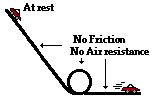a. A Hot Wheels car starts from rest on top of an inclined plane and rolls down the incline through a loop and along a horizontal surface. Ignore the effect of friction and air resistance on the car.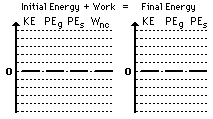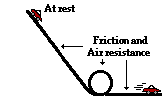b. A Hot Wheels car starts from rest on top of an inclined plane and rolls down the incline through a loop and along a horizontal surface. Friction and air resistance have a significant effect on the car.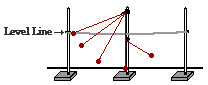c. A pendulum bob is mounted on top of a lab pole and drawn back to a string which it tied between two other poles. The pendulum bob is released from rest. Upon reaching the vertical, the string hits a barrier and a new pivot point is established as the bob continues in motion along an upward trajectory. Ignore the effect of air resistance.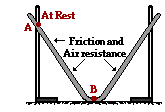d. A track is constructed by stretching a grooved and pliable material between two lab poles. A metal ball starts from rest at point A and rolls along the groove to point B. Friction and air resistance have an effect upon the ball's motion.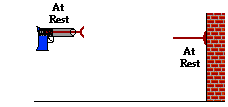e. A dart is placed in a dart gun and the springs are compressed. The trigger is pulled and the dart is projected towards a wall. The dart collides with the wall and sticks to it. Ignore the effect of friction and air resistance.f. Ben Laborin applies a force to push a crate from the bottom of an inclined plane to the top at a constant speed.36. Several physical situations are described below. For each situation, simplify the work-energy equation by canceling any zero terms and any energy terms (whether KE or PE) which are unchanging. Explain each term which gets canceled. The first problem is done as an example.

 Description of Physical Situation Simplification of Work-Energy Equation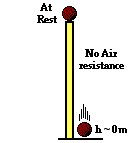a. A ball starts from rest on top of a tall pillar and falls to the ground below. Assume the effect of air resistance is negligible. KEi + PEi + Wnc = KEf + PEf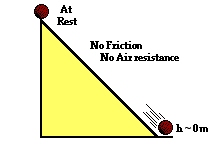b. A ball starts from rest at an elevated position along an inclined plane and rolls to the ground below. Assume that the effect of friction and air resistance is negligible. KEi + PEi + Wnc = KEf + PEf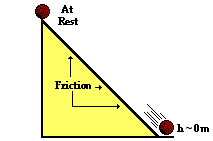c. A ball starts from rest at an elevated position along an inclined plane and rolls to the ground below. Consider the effect of friction and air resistance. KEi + PEi + Wnc = KEf + PEf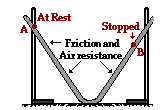d. A track is constructed by stretching a grooved and pliable material between two lab poles. A metal ball starts from rest at point A and rolls to point B. Friction and air resistance have an effect upon the ball's motion. KEi + PEi + Wnc = KEf + PEfe. A pendulum bob is mounted on top of a lab pole and drawn back to a string which it tied between two other poles. The pendulum bob is released from rest. Upon reaching the vertical, the string hits a barrier and a new pivot point is established as the bob continues in motion along an upward trajectory. Ignore the effect of air resistance. KEi + PEi + Wnc = KEf + PEff. A Hot Wheels car starts from rest on top of an inclined plane and rolls down the incline through a loop and along a horizontal surface. Friction and air resistance have a significant effect on the car. KEi + PEi + Wnc = KEf + PEf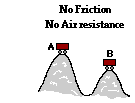g. An unattended hot dog wagon starts from rest and rolls down a hill and up a second hill. Ignore the effect of friction and air resistance. KEi + PEi + Wnc = KEf + PEfh. A roller coaster car is already in motion on the top of the first drop and rolls along the track over a couple of hills. Ignore the effect of friction and air resistance. KEi + PEi + Wnc = KEf + PEf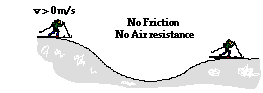i. A cross-country skier is in motion on top of a small hill. He skis down the hill into the valley and up a second smaller hill. He uses his poles to propel himself during the entire motion. Ignore the effect of friction and air resistance. KEi + PEi + Wnc = KEf + PEf

### Part D: Complex Analysis and Problem-Solving

37. A 21.3-kg child positions himself on an inner-tube which is suspended by a 7.28-m long rope attached to a strong tree limb. The child and tube is drawn back until it makes a 17.4-degree angle with the vertical. The child is released and allowed to swing to and from. Assuming negligible friction, determine the child's speed at his lowest point in the trajectory. PSYW

38. A baseball player catches a 163-gram baseball which is moving horizontally at a speed of 39.8 m/s. Determine the force which she must apply to the baseball if her mitt recoils a horizontal distance of 25.1 cm. PSYW

39. A 62.9-kg downhill skier is moving with a speed of 12.9 m/s as he starts his descent from a level plateau at 123-m height to the ground below. The slope has an angle of 14.1 degrees and a coefficient of friction of 0.121. The skier coasts the entire descent without using his poles; upon reaching the bottom he continues to coast to a stop; the coefficient of friction along the level surface is 0.623. How far will he coast along the level area at the bottom of the slope? PSYW

40. A 29.1-kg sledder is traveling along a level area with a speed of 8.96 m/s when she approaches a gentle incline which makes an angle of 12.5 degrees with the horizontal. If the coefficient of friction between the sled and the incline is 0.109, then what will be her speed at the bottom of the inclined plane, located 8.21 m above the top of the incline. PSYW

41. A 221-gram ball is thrown at an angle of 17.9 degrees and a speed of 36.7 m/s from the top of a 39.8-m high cliff. Determine the impact speed of the ball when it strikes the ground. Assume negligible air resistance. PSYW

42. Claire deAisles has just completed her shopping at the grocery food store. She accidentally bumps her 42.5-kg cart, setting it in motion from rest down a hill inclined at 14.9 degrees. Upon descending a distance of 9.27 meters along the inclined plane, the cart hits a tree stump (which was placed in the parking lot for the sole purpose of this problem). A 0.295-kg can of tomato soup is immediately hurled from the moving cart and heads towards Will N. Tasue's brand new Lexus. Upon striking the Lexus, the tomato soup can creates a dent with a depth of 3.16 cm. Noah Formula, who is watching the entire incident and fixing to do some physics, attempts to calculate the average force which the Lexus applies to the soup can. Assume negligible air resistance and friction forces and help Noah out. PSYW

43. Pete Zaria applies a 11.9-Newton force to a 1.49-kg mug of root beer in order to accelerate it from rest over a distance of 1.42-m. Once released, how far will the mug slide along the counter top if the coefficient of friction is 0.728? PSYW

44. Suzie Lovtaski has a mass of 49.7 kg. She is at rest on top of a hill with a height of 92.6 m and an incline angle of 19.2 degrees. She coasts down the hill to the bottom and eventually comes to a stop; she never uses her poles to apply a force. The coefficient of friction is 0.0873 along the hill and 0.527 along the horizontal surface at the bottom. What total distance will Suzie coast (include both incline and level surface)? PSYW

45. Mia Kneezhirt jumps from a second story dorm room (h = 7.91 m) to the ground below. Upon contact with the ground, she allows her 62.4-kg body to come to an abrupt stop as her center of gravity is displaced downwards a distance of 89.2 cm. Calculate the average upward force exerted by the ground upon Mia's fragile body. PSYW

#### Navigate to:

Review Session Home - Topic Listing

Work and Energy - Home || Printable Version || Questions with Links

Answers to Questions:  All || #1-10 || #11-25 || #26-36 || #37-45

## You Might Also Like ...

Users of The Review Session are often looking for learning resources that provide them with practice and review opportunities that include built-in feedback and instruction. If that is what you're looking for, then you might also like the following:

The Calculator Pad includes physics word problems organized by topic. Each problem is accompanied by a pop-up answer and an audio file that explains the details of how to approach and solve the problem. It's a perfect resource for those wishing to improve their problem-solving skills.

Visit: The Calculator Pad Home | Calculator Pad - Work and Energy

2. Minds On Physics the App Series

Minds On Physics the App ("MOP the App") is a series of interactive questioning modules for the student that is serious about improving their conceptual understanding of physics. Each module of the series covers a different topic and is further broken down into sub-topics. A "MOP experience" will provide a learner with challenging questions, feedback, and question-specific help in the context of a game-like environment. It is available for phones, tablets, Chromebooks, and Macintosh computers. It's a perfect resource for those wishing to refine their conceptual reasoning abilities. Part 3 of the series includes topics in Work and Energy.

Visit: MOP the App Home || MOP the App - Part 3